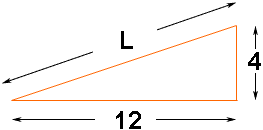Quandaries and Queries According to my study material 4:12 multiplying factor for shingles is 1.054.  The question reads as follows:  A building with a floor plan of 3350 sq. ft. and a roof slope of 4:12 will require _______ bundles of standard asphalt shingles.   Please show me how to find the multiplying factor 1.054 from 4:12 roof slope.   Thanks again. Hi Larry, A roof slope of 4:12 means that in a "run" of 12 units there is a "rise" of 4 units as in the diagram below.The triangle in the diagram ia a right triangle so, by Pythagoras' Theorem, L2 = 122 + 42 = 114 + 16 = 160 Thus L is the square root of 160 which is 12.6491.  12.6491/12 = 1.054 and hence the length of L is 1.054 times the run. Harley Go to Math Central